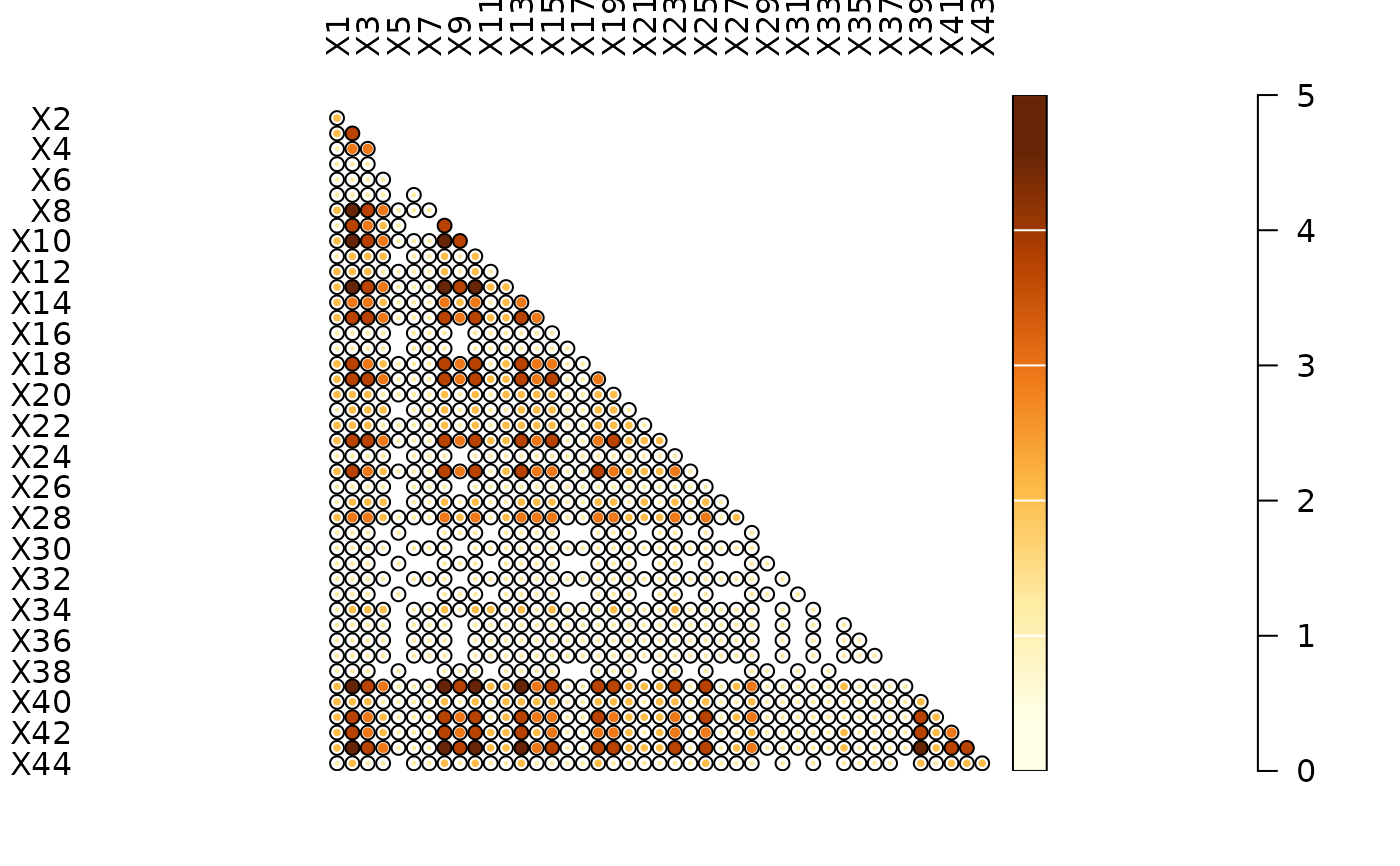Co-Occurrence

## Usage

occurrence(object, ...)

# S4 method for matrix
occurrence(object)

# S4 method for data.frame
occurrence(object)

## Arguments

object

A $$m \times p$$ matrix of count data.

...

Currently not used.

## Value

A stats::dist object.

## Details

A co-occurrence matrix is a symmetric matrix with zeros on its main diagonal, which works out how many times each pairs of taxa/types occur together in at least one sample.

Other diversity measures: heterogeneity(), plot_diversity, rarefaction(), richness(), similarity(), simulate(), turnover()

N. Frerebeau

## Examples

## Plot spot diagram of a co-occurrence matrix
data("mississippi", package = "folio")

occ <- occurrence(mississippi)
plot_spot(occ)